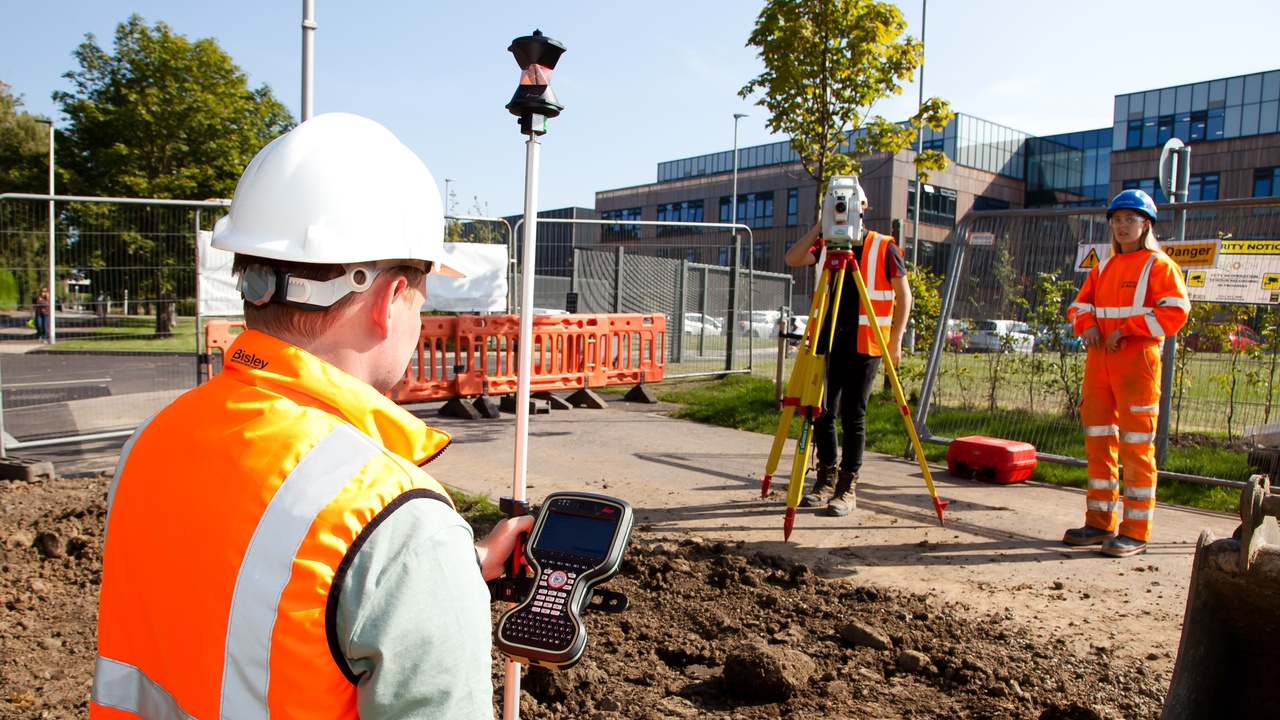# Setting Out For Construction

### What you'll get:

Levelling and Taping;

• The principal of levelling
• Levelling terminology
• Setting up the level
• Parallax
• Staff verticality
• Checking and adjusting the pod bubble
• Principal of the 2 peg test
• Two peg test booking example
• Level survey principal
• Level survey booking example
• Transferring a TBM principal
• Transferring a TBM booking example
• Transferring a TBM calculations and checks
• Inverted staff reading booking example
• Setting out levels
• Checking levels
• Profile boards for level excavation principal
• Profile boards for sloped excavation principal
• Profile boards for drainage run preparation calculations
• Profile boards for drainage run booking example
• Profile boards for drainage run demonstration
• Batter rails principal
• Batter rails for an excavation tutorial
• Batter rails for fill tutorial
• Setting up a laser level

Total Station

• Introduction to the total station
• Setting up over a point
• Best practice observing
• Calibration certificate
• Putting it into the box
• Collimation checks - Observing
• Horizontal collimation error booking example
• Vertical collimation error booking example
• Diaphragm orientation check
• Traverse principal
• Traverse booking example
• Traverse whole circle bearing
• Traverse table
• Resection
• Volume of a stockpile
• Reference line/reference arc
• Instruction for a resection (Leica TS06 or TS09)
• Instructions for stakeout function (Leica TS06 or TS09)
• Exporting data from the total station
• Importing data into the total station
• Setting up control points for practice exercises

Maths: Basic angles, distances and coordinates

• Outline and objectives
• Introduction to Pythagoras
• Pythagoras (how to calculate the diagonal of a rectangle or triangle)
• Pythagoras - Worked example
• Calculator short cut for Pythagoras
• Quiz 1: Pythagoras
• Introduction to angles
• Convert decimal angles to degrees, minutes and seconds
• Quiz 2: angles
• Trigonometry basics
• Enter INVERSE sin, cos, tan in your calculator
• Quiz 3: Trigonometry
• Introduction to coordinates
• Distance between two coordinates - worked example
• Quiz 4: Coordinates
• Introduction to Whole circle bearings
• WCB worked example 1
• WCB worked example 2
• WCB worked example 3
• WCB worked example 4
• Quiz 5: WCB
• Test yourself
• Summary